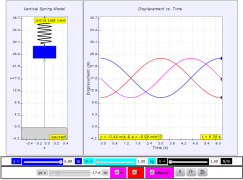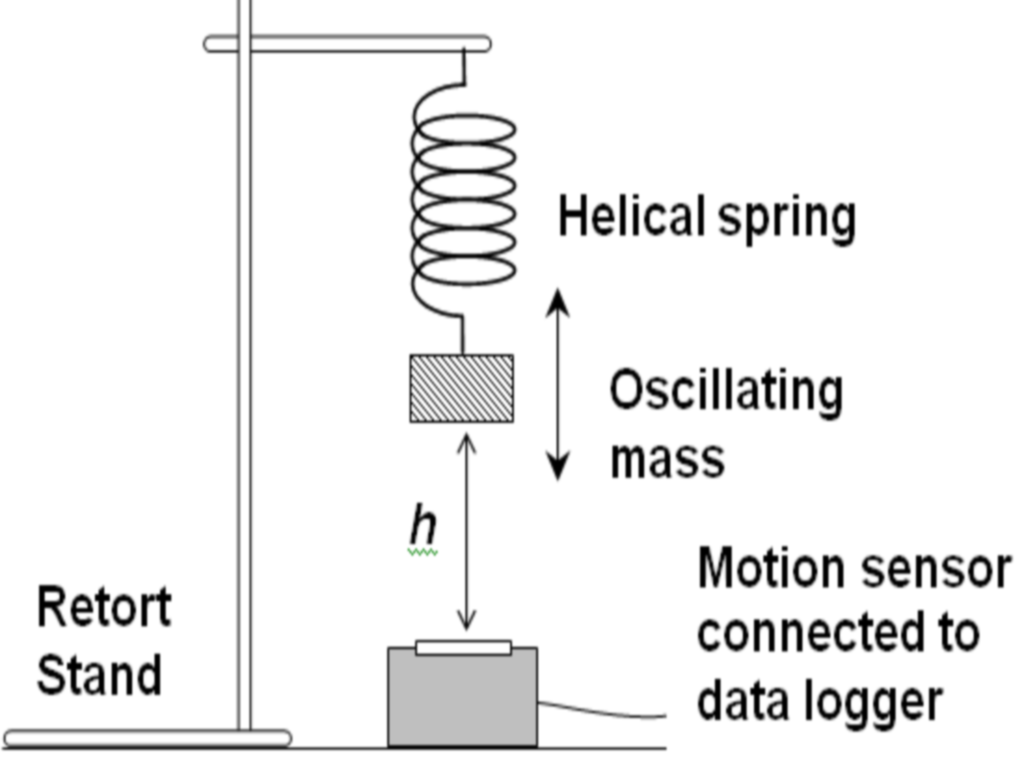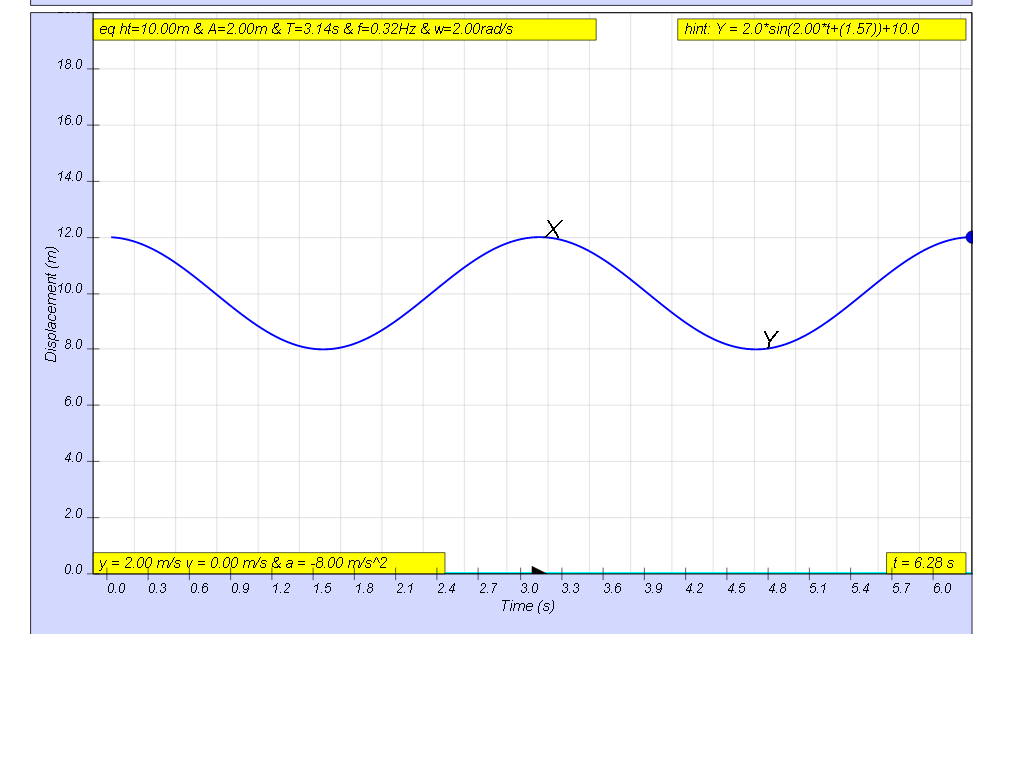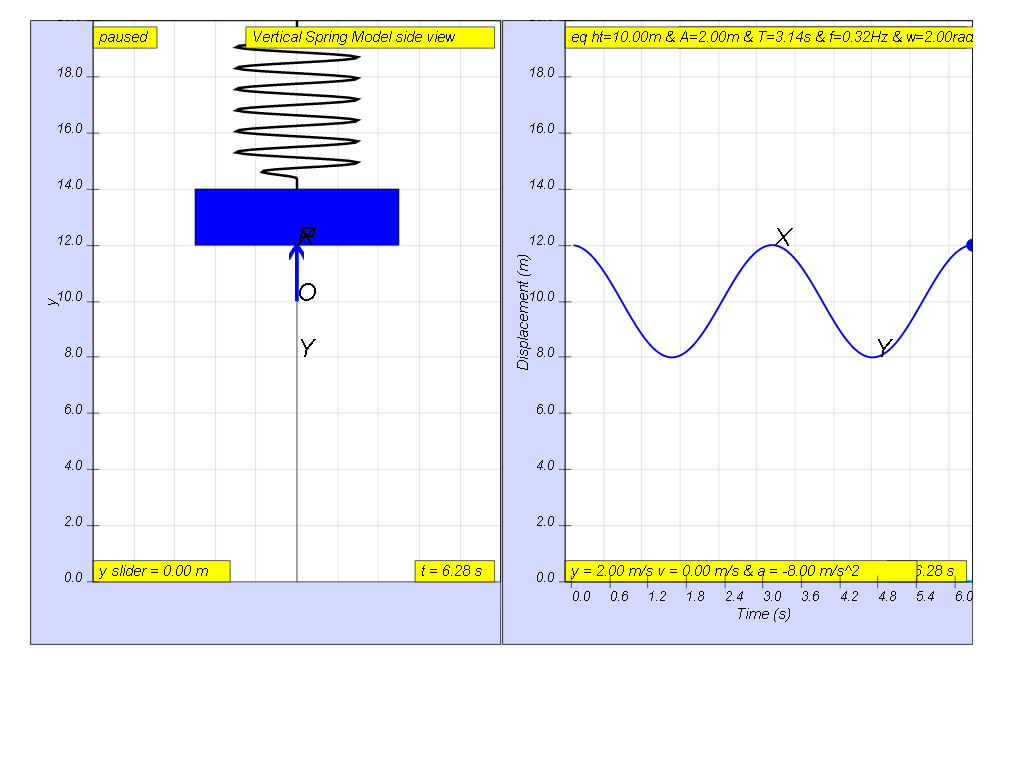SHM06

### 1.2.13 Experimental Investigation of Vertical Oscillator              LO (b)

Here is an illustration of how the oscillation of a basic spring-mass system can be investigated using motion sensor.1. Setup the experiment as shown
2. Displace the mass vertically downwards and release it with initial velocity =0 m/s so that it can oscillate freely in an up and down motion.
3. Start the data logger when the oscillations are steady.
4. The motion sensor detects the variation with time of height h.

Example of data collected is shownInformation of the oscillation can be drawn from Fig. 13.14 as follows:

1. Equilibrium height         h    =
2. Amplitude            x0     =
3. Period                T     =
4. Frequency            f    =
5. Angular frequency        ω     =
6. Displacement when t = 5.7 s    x    =

[ 10.0 m , 2.0 m , 3.14 s , 0.32 Hz, 2.00 rad/s, +1.0 m)### 1.2.13.1 Model:

Q1: setup the model to represent the conditions in the example hint: select sensor?,  y = 2.0m, k = 4 N/m   etc.

Q2: setup the model with different conditions of your choice and recalculate the point 1 to 6.

Q3: a student suggests that the conditions of getting the same period of  T = 3.14 s is to ensure the following equation is valid.

$\sqrt{\frac{m}{k}}=\frac{1}{2}$collect some data on your own, to verify this claim by this student. Suggest with reasons, whether the claim is supported by the evidences you can collect from the model.

### Translations

Code Language Translator Run### Software Requirements

SoftwareRequirements

 Android iOS Windows MacOS with best with Chrome Chrome Chrome Chrome support full-screen? Yes. Chrome/Opera No. Firefox/ Samsung Internet Not yet Yes Yes cannot work on some mobile browser that don't understand JavaScript such as..... cannot work on Internet Explorer 9 and below

### CreditsThis email address is being protected from spambots. You need JavaScript enabled to view it.

### end faq

http://iwant2study.org/lookangejss/02_newtonianmechanics_8oscillations/ejss_model_SHM163/SHM163_Simulation.xhtml

3.5 1 1 1 1 1 1 1 1 1 1 Rating 3.50 (3 Votes)